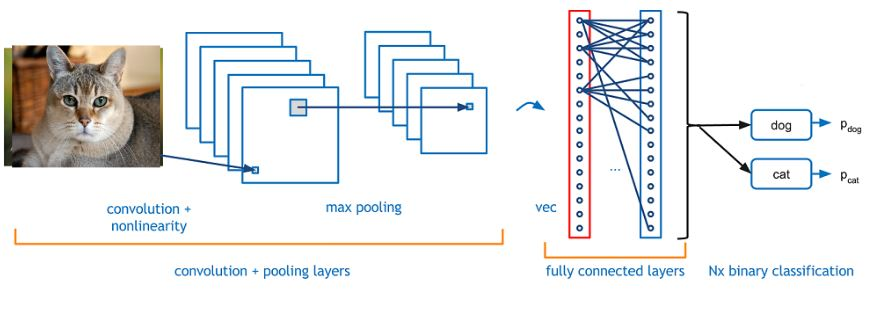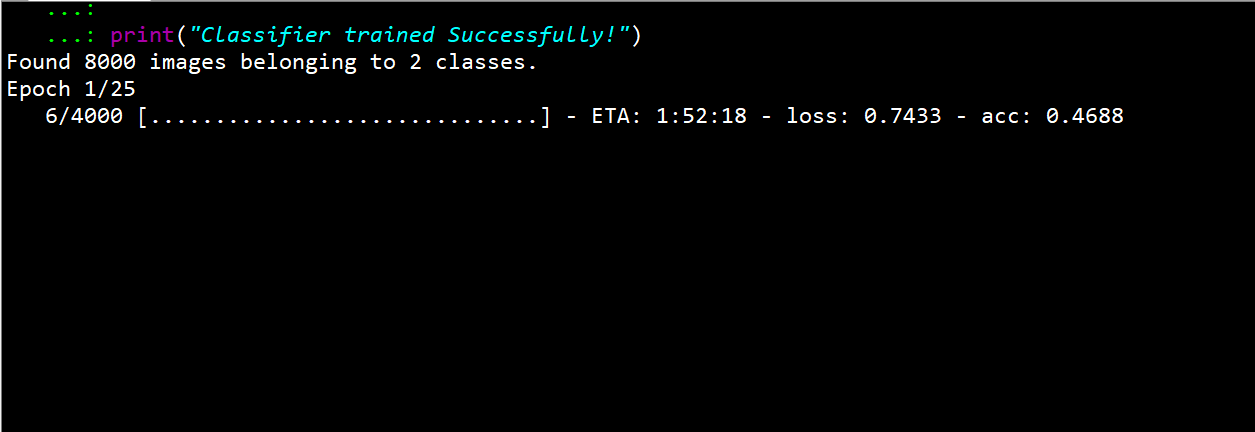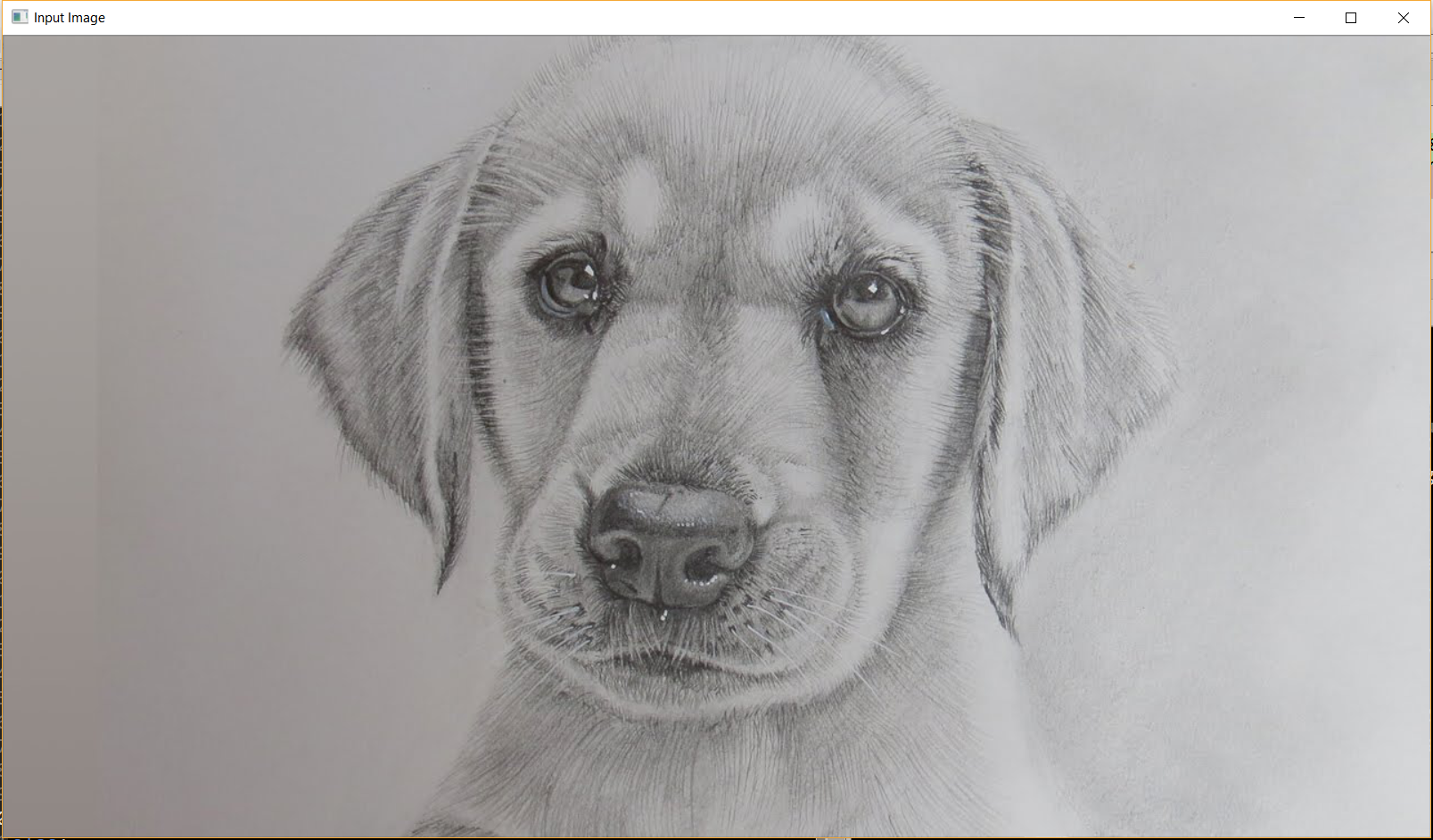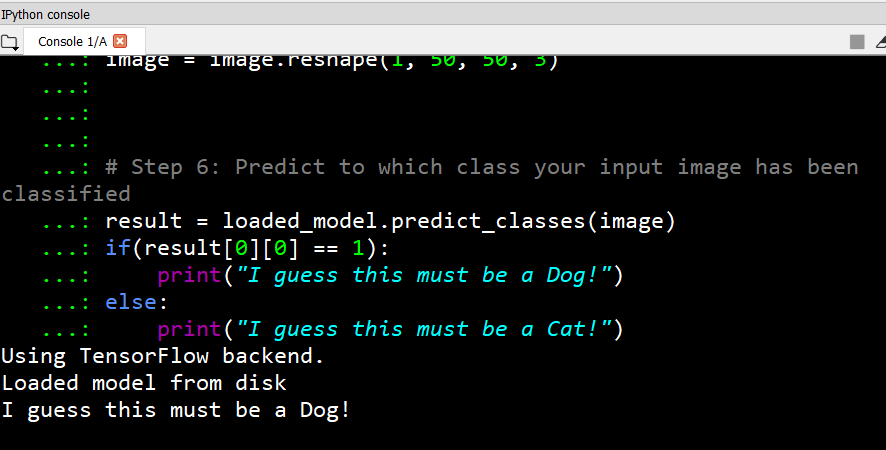# Project Idea | Cat vs Dog Image Classifier using CNN implemented using Keras

• Difficulty Level : Basic
• Last Updated : 09 Jul, 2021

Project Title: Cat vs Dog Image Classifier

Introduction:

This project aims to classify the input image as either a dog or a cat image. The image input which you give to the system will be analyzed and the predicted result will be given as output. Machine learning algorithm [Convolutional Neural Networks] is used to classify the image.
The model thus implemented can be extended to a mobile device or any website as per the developer’s need.

You can get the complete code for the project here

Conceptual Framework:
The project is entirely implemented using Python3. The Conceptual Framework involved is mainly:

• Keras – Tensorflow backend
• OpenCV – Used to handle image operationsMethod:

Step 1: Getting the Dataset

The dataset is available here

Step 2: Installing Required Packages [Python 3.6]

1. OpenCV —> ‘3.4.0’ [ Used to handle image operations like reading the image, resizing, reshaping]
2. numpy —> ‘1.14.4’ [ Image that is read will be stored in an numpy array ]
3. TensorFlow —> ‘1.8.0’ [ Tensorflow is the backend for Keras ]
4. Keras —> ‘2.1.6’ [ Keras is used to implement the CNN ]

Step 3: How the Model Works ??

The dataset contains a lot of images of cats and dogs. Our aim is to make the model learn the distinguishing features between the cat and dog. Once the model has learned, i.e once the model got trained, it will be able to classify the input image as either cat or a dog.

Features Provided:

• Own image can be tested to verify the accuracy of the model
• This code can directly be integrated with your current project or can be extended as a mobile application or a site.
• To extend the project to classify different entities, all you need to do is find the suitable dataset, change the dataset accordingly and train the model

Diagram/Output :Data structures and Algorithms used in project

• Numpy Array: This most powerful and widely used data structure of python is used to store the pixel value of images.

Tools Used:

• Python Interpreter
• Anaconda Prompt
• Spyder

Applications:
This project gives a general idea of how image classification can be done efficiently. The scope of the project can be extended to the various industries where there is a huge scope for automation, by just altering the dataset which is relevant to the problem.

My Personal Notes arrow_drop_up